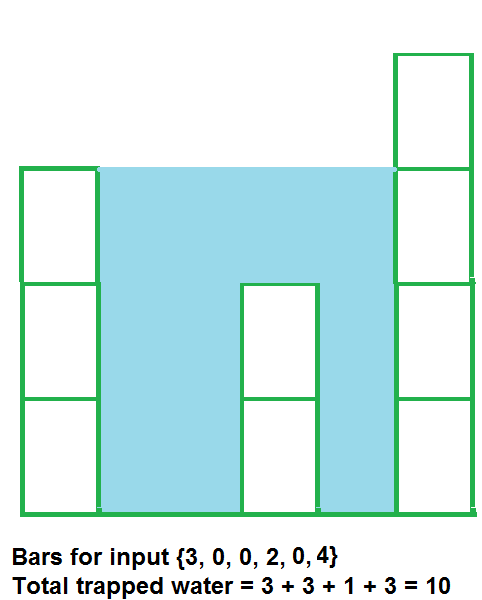Trapping Rain Water
Medium Accuracy: 49.62% Submissions: 91662 Points: 4

Given an array arr[] of N non-negative integers representing the height of blocks. If width of each block is 1, compute how much water can be trapped between the blocks during the rainy season.

Example 1:

Input:
N = 6
arr[] = {3,0,0,2,0,4}
Output:
10
Explanation:Example 2:

Input:
N = 4
arr[] = {7,4,0,9}
Output:
10
Explanation:
Water trapped by above
block of height 4 is 3 units and above
block of height 0 is 7 units. So, the
total unit of water trapped is 10 units.


Example 3:

Input:
N = 3
arr[] = {6,9,9}
Output:
0
Explanation:
No water will be trapped.

You don't need to read input or print anything. The task is to complete the function trappingWater() which takes arr[] and N as input parameters and returns the total amount of water that can be trapped.

Expected Time Complexity: O(N)
Expected Auxiliary Space: O(N)

Constraints:
3 < N < 106
0 < Ai < 108

We are replacing the old Disqus forum with the new Discussions section given below.

### Editorial

We strongly recommend solving this problem on your own before viewing its editorial. Do you still want to view the editorial?

#### My Submissions: